SEARCH HOMEMath Central Quandaries & QueriesQuestion from Lauren, a student: How do you convert eleven fifteenths into a decimal?Lauren, we have two responses for you

Lauren,

To convert 11/15 into a decimal divide 15 into 11.

Penny

Hi Lauren. I don't like doing long division. It's important to know how to do it, but sometimes there are ways to avoid it if you don't like it.

Do you know how to convert one third into a decimal?

Sometimes we can make simpler fractions even from fractions like 11/15 which already seem like they are in the simplest form.

For this question, I'm going to multiply 11/15 by ten and also divide it by ten. When I do that to any number, it doesn't really change.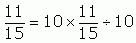I know that multiplying a fraction by a whole number means just multiplying the whole number on the numerator (top) of the fraction.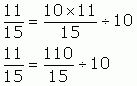Because 110 is not smaller than 15, we call 110/15 an "improper" fraction. That's okay, but it also means I can change it into a "mixed" fraction. I know that 15 goes into 110 7 times with remainder 5, so that means 110/15 is the mixed fraction seven and 5/15. And I bet you can see that you can reduce 5/15 into 1/3 by dividing the top and the bottom each by 5.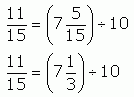You probably already know the simple fraction 1/3 is 0.33333... repeating, so 7 and 1/3 is 7.33333.... repeating: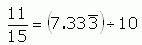Last step: dividing by ten is easy with decimal numbers, because we just more the decimal place one position to the left. In this case, that means moving it from in front of the first 3 to in front of the 7: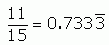And that's another way to solve the problem. Long division is probably faster in this question, because you can quickly find that the three repeats, but if you really don't like long division, there are sometimes other ways to solve the questions. Math is like that: there are usually lots of ways of solving a question, but some are harder than others, so it helps to know different methods you can try.

Cheers,
Stephen La Rocque.Math Central is supported by the University of Regina and The Pacific Institute for the Mathematical Sciences.